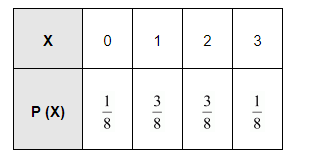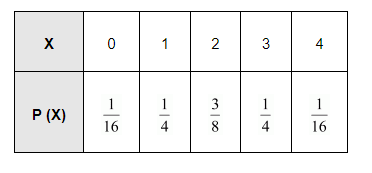# Find the probability distribution of

Question:

Find the probability distribution of

(i) number of heads in two tosses of a coin

(ii) number of tails in the simultaneous tosses of three coins

(iii) number of heads in four tosses of a coin

Solution:

(i) When one coin is tossed twice, the sample space is

{HH, HT, TH, TT}

Let X represent the number of heads.

∴ X (HH) = 2, X (HT) = 1, X (TH) = 1, X (TT) = 0

Therefore, X can take the value of 0, 1, or 2.

It is known that,

$\mathrm{P}(\mathrm{HH})=\mathrm{P}(\mathrm{HT})=\mathrm{P}(\mathrm{TH})=\mathrm{P}(\mathrm{TT})=\frac{1}{4}$

$P(X=0)=P(T T)=\frac{1}{4}$

$P(X=1)=P(H T)+P(T H)=\frac{1}{4}+\frac{1}{4}=\frac{1}{2}$

$\mathrm{P}(\mathrm{X}=2)=\mathrm{P}(\mathrm{HH})=\frac{1}{4}$

Thus, the required probability distribution is as follows.(iii) When a coin is tossed four times, the sample space is

$\mathrm{S}=\left\{\begin{array}{c}\mathrm{HHHH}, \mathrm{HHHT}, \mathrm{HHTH}, \mathrm{HHTT}, \mathrm{HTHT}, \mathrm{HTHH}, \mathrm{HTTH}, \mathrm{HTTT}, \\ \mathrm{THHH}, \mathrm{THHT}, \mathrm{THTH}, \mathrm{THTT}, \mathrm{TTHH}, \mathrm{TTHT}, \mathrm{TTTH}, \mathrm{TTTT}\end{array}\right\}$

Let X be the random variable, which represents the number of heads.

It can be seen that X can take the value of 0, 1, 2, 3, or 4

$P(X=0)=P(T T T T)=\frac{1}{16}$

P (X = 1) = P (TTTH) + P (TTHT) + P (THTT) + P (HTTT)

$=\frac{1}{16}+\frac{1}{16}+\frac{1}{16}+\frac{1}{16}=\frac{4}{16}=\frac{1}{4}$

P (X = 2) = P (HHTT) + P (THHT) + P (TTHH) + P (HTTH) + P (HTHT)

+ P (THTH)

$=\frac{1}{16}+\frac{1}{16}+\frac{1}{16}+\frac{1}{16}+\frac{1}{16}+\frac{1}{16}=\frac{6}{16}=\frac{3}{8}$

P (X = 3) = P (HHHT) + P (HHTH) + P (HTHH) P (THHH)

$=\frac{1}{16}+\frac{1}{16}+\frac{1}{16}+\frac{1}{16}=\frac{4}{16}=\frac{1}{4}$

$P(X=4)=P(\mathrm{HHHH})=\frac{1}{16}$

Thus, the probability distribution is as follows.